CipherJan 22, 2017

Artist protests Trump with bloody mural - Assassination codes

Once again we are receiving assassination coding for Trump, and there's many interesting things with this post

Here is the CNN's article - http://edition.cnn.com/2017/01/18/arts/protesting-trump-illma-gore-blood-mural/index.html

My prediction is for May First 2017, I have 3 posts explaining why that date fits perfect with all of the coding, as I will show again in this post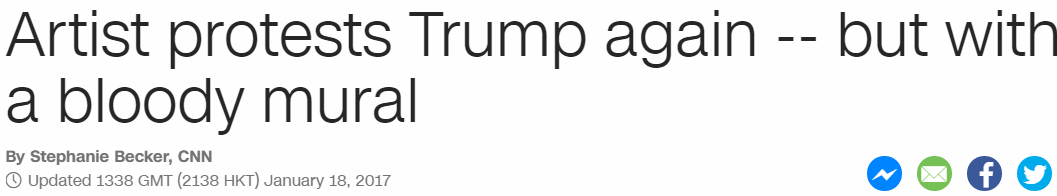This was released on January 18th, 2017 - 1/18
Death = 4+5+1+100+8 = 118 (Jewish)

1/18/2017 - 1+18+20+17 = 56
president = 7+9+5+10+9+4+5+5+2 = 56 (S Exception)

Now this date is interesting because it is a perfect match with 11/9/2016 when Trump won the election
11/9/2016 - 1+1+9+2+0+1+6 = 20
11/9/2016 - 11+9+2+0+1+6 = 29
11/9/2016 - 11+9+20+16 = 56
11/9/16 - 11+9+16 = 36 (36th triangular number is 666, 'May First' = 666 - Sumerian)

1/18/2017 - 1+1+8+2+0+1+7 = 20
1/18/2017 - 1+18+2+0+1+7 = 29
1/18/17 - 1+18+17 = 36

Donald Trump = 4+6+5+1+3+4+2+9+3+4+7 = 48 (Reduced)
Donald Trump = 4+15+14+1+12+4+20+18+21+13+16 = 138 (Ordinal)
138+831 = 969, the Speed of Saturn is 969 km/s
Bloody Mural = 2+3+6+6+4+7+4+3+9+1+3 = 48 (Reduced)
Bloody Mural = 2+12+15+15+4+25+13+21+18+1+12 = 138 (Ordinal)

The reason that they say Artists protests again is because on March 27th 2016 artist Brian Andrew Whiteley dropped off Trump's headstone in 'Central Park' = 119 (Ordinal)
Very important to note, the Tombstone was dropped off 227 days before 11/9 when Trump won the election and November 9th is 314th day in the year (22/7 = 3.14)

Brian = 2+18+9+1+14 = 44 (Ordinal)
Whiteley = 5+8+9+2+5+3+5+7 = 44 (Reduced)
Kill = 44, Execution = 44, Genocide = 44, Military = 44... Important to note that Brian was 33 years old at the time2016 was a leap year and 3/27 was 87th day
Eight Seven = 5+9+7+8+100+90+5+700+5+40 = 969 (Jewish)
Twenty seventh of March = 2+5+5+5+2+7+1+5+4+5+5+2+8+6+6+4+1+9+3+8 = 93 (Reduced)
Saturn = 19+1+20+21+18+14 = 93 (Ordinal)Artist uses real blood to protest trump = 1+9+2+9+1+2+3+1+5+1+9+5+1+3+2+3+6+6+4+2+6+7+9+6+2+5+1+2+2+9+3+4+7 = 138 (Reduced)
Donald Trump = 4+15+14+1+12+4+20+18+21+13+16 = 138 (Ordinal)
138+831 = 969
Divisors of 969 sum to 1440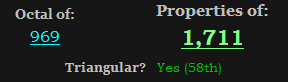Trump just entered office on 58 date numerology, Trump's Inaugural ceremony was lead by 58 year old Steve Ray
Steve Ray = 19+20+5+22+5+18+1+25 = 115 (Ordinal)
Fifty-eight = 6+9+6+20+25+5+9+7+8+20 = 115 (Ordinal)
In the video they say that there is over 55 donors
fifty five donors = 6+9+6+20+25+6+9+22+5+4+15+14+15+18+19 = 193 (Ordinal)
44th prime number is 193 (Kill = 44)
Over fifty five donors = 6+4+5+9+6+9+6+2+7+6+9+4+5+4+6+5+6+9+10 = 118 (S Exception)
Death = 118 (Jewish)
Over fifty five donors = 60+400+5+90+6+9+6+200+700+6+9+400+5+4+60+50+60+90+100 = 2260 (English)
 Two hundred twenty-six = 2+5+6+8+3+5+4+9+5+4+2+5+5+5+2+7+1+9+6 = 93 (Reduced)

The video that CNN gave us today is 1 minute and 33 seconds long which is 93 seconds
President = 11+9+22+8+18+23+22+13+7 = 133 (Reverse Ordinal)
There are 3 ways to order the number 133..... 331+313+133 = 777, also when you write out 133 and 331 they have the same Jewish gematria of 1331
Saturn = 90+1+100+200+80+40 = 511 (Jewish)
511+151+115 = 777 (Trump ran for 511 days, won on a date numerology of 36 (36th prime is 151) and he went into office with 115th Congress on a date numerology of 58, Fifty-eight = 115)
Cube of Saturn = 3+200+2+5+50+6+90+1+100+200+80+40 = 777 (Jewish)
Order out of Chaos = 50+80+4+5+80+50+200+100+50+6+3+8+1+50+90 = 777 (Jewish)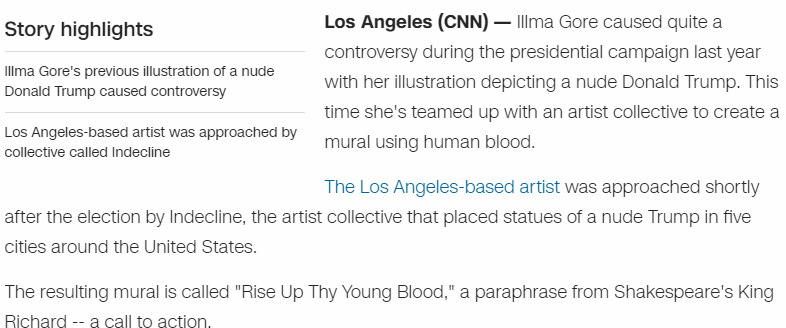This was painted by Illma GoreHer real name has extremely interesting gematria
Ashley Gore = 1+19+8+12+5+25+7+15+18+5 = 115 (Ordinal)
Ashley Gore = 1+90+8+20+5+400+7+50+80+5 = 666 (Jewish)
May First = 78+6+150+36+54+108+114+120 = 666 (Sumerian)

Illma Gore = 9+3+3+4+1+7+6+9+5 = 47 (Reduced)
President = 7+9+5+1+9+4+5+5+2 = 47 (Reduced)Indecline = 9+5+4+5+3+3+9+5+5 = 48 (Reduced)
Bloody Mural = 2+3+6+6+4+7+4+3+9+1+3 = 48 (Reduced)

Indecline = 9+40+4+5+3+20+9+40+5 = 135 (Jewish)
531+135 = 666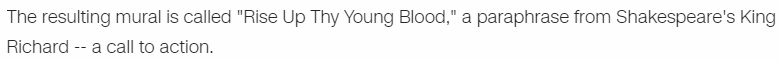Rise Up Thy Young Blood = 18+9+19+5+21+16+20+8+25+25+15+21+14+7+2+12+15+15+4 = 271 (Ordinal)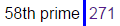This was the 58th US election and Trump just entered office on a date with 58 numerology

If Trump dies on 5/1 it will be 44 days before his 71st birthday
Illma Gore = 35+12+12+13+1+33+15+18+5 = 144 (Francis Bacon)
Forty four = 6+15+18+20+25+6+15+21+18 = 144 (Ordinal)
Seventy-one = 19+5+22+5+14+20+25+15+14+5 = 144 (Ordinal)

This came out on 71st day after Trump won the electionThere's so much I documented in the previous 3 days with Trump assassination codes, I guess we'll see what happens, hopefully I didn't prevent the assassination haha

1 comment:

1.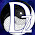Great article! I mean it. You do great decoding. The Trump Building is 71-storeys tall. There's an article on my blog about the "Flag Hijab" mentions that. The "blood mural" is sick and twisted. It reminds me of their spirit cooking ritual.